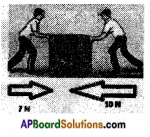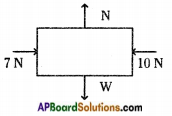AP State Syllabus AP Board 8th Class Physical Science Important Questions Chapter 1 Force

## AP State Syllabus 8th Class Physical Science Important Questions 1st Lesson Force

### 8th Class Physical Science 1st Lesson Force 1 Mark Important Questions and Answers

Question 1.
What is force ? What is its S.I. unit?
The push or pull of an object is called force. Its S.I. unit is Newton.

Question 2.
What is a contacting force?
The force results when there is a direct physical contact between two interacting objects is called contacting force.Question 3.
Define a force at a distance or field force.
The force which occurs without any physical contact between two objects is known as force at a distance or field force.

Question 4.
What is muscular force?
The force which we exert by using our body muscles is known as muscular force.

Question 5.
What is friction?
The resistance to the movement of a body over the surface of another body is called friction.

Question 6.
Define normal force.
The force that a solid surface exerts on any object in the normal direction is called normal force.

Question 7.
What is a magnetic force?
The force exerted by a magnet on another magnet.

Question 8.
What is a gravitational force?
The attractive forces between any two massive objects is called gravitational force.Question 9.
What is electrostatic force?
The force exerted by a charged body on other charged or uncharged body is called electrostatic force.

Question 10.
What is magnetic field?
The space around the magnet where its influence can be detected is called magnetic field.

Question 11.
What is a net force?
The algebraic sum of the forces acting on a body is known as net force.

Question 12.
What is free body diagram?
The diagram showing all the forces acting on an object at a particular instant is called free body diagram.

Question 13.
A blacksmith hammers a hot piece of iron while making a tool. How does the force due to hammering affect the piece of iron?
It changes the shape of iron.

Question 14.
A rocket has been fired upwards to launch a satellite in its orbit. Name the two forces acting on the rocket immediately after leaving the launching pad?

1. The weight of the rocket downwards.
2. The upward force due to firing of rocket.

Question 15.
Why do not you experience gravitational force exists between you and your teacher because gravitational force depends on masses of object
The reason is everything on earth experienced by a large gravitational force of earth so remaining gravitational forces are negligible.Question 16.
What is tension?
When we stretch a rope or a string the tightness of rope or string is called tension. Tension is a contact force.

### 8th Class Physical Science 1st Lesson Force 2 Marks Important Questions and Answers

Question 1.
Explain Gravitational Force by giving a suitable example.
Gravitational Force: The force of attraction that exists between any two bodies (or masses) everywhere in the universe.
Examples:

1. The stone thrown up into the sky fall back on to the earth due to gravitational force.
2. Earth holding atmosphere due to gravitational force.
3. All the objects are pulling towards earth due to gravitational force.
4. Rivers flow down to the sea because of gravitational force.

Question 2.
The surface area of an object is 20 m2 and a force of 10 N is applied on it, then what is the pressure?
Force (F) = 10 N; Area (A) = 20 m × 2m = 40m2;
Pressure = $$\frac{F}{A}$$ = $$\frac{10}{40}$$ = 0.25 Pascal

Question 3.
Observe the situations of electrostatic forces in your daily life and prepare a report.

1. If you comb your dry hair and bring small pieces of paper then these paper pieces are attracted by comb because it is charged.
2. If a glass rod is rubbed with a silk cloth then if we bring small pieces of paper near glass rod or silk cloth then these paper pieces are attracted by glass rod or silk cloth due to elctrostatic force.Question 4.
Find the net force acting on a body shown in the figure. And draw the direction of net force. (Length of the arrow shows the magnitude of the force and direction also.)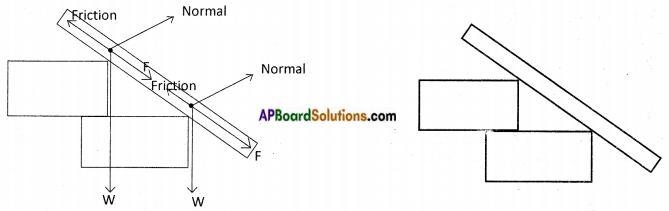Question 5.
Give two daily life examples each of situations in which you push or pull to change the state of motion of object.
Push:

1. Hitting a foot ball with your leg.

Pull:

1. Opening a door
2. Moving a table towards you.

Question 6.
Give two examples of situations in which applied force causes a change in the shape of an object.

1. Pressing a sponge.
2. Stretching of rubber band.

Question 7.
Name the forces acting on a plastic bucket containing water held above ground level in your hand. Why do the forces acting on the bucket do not bring a change in its state of motion?
The gravitational force acts as plastic bucket containing water held above ground level in your hand. Gravitational force does not bring change in state of bucket be¬cause equal amount of force is applied vertically upward.

Question 8.
Name the two forces acting on a rocket immediately after leaving the launching pad.
The two forces acting on rocket are:

1. Force due to gravity vertically downward.
2. Frictional force of atmosphere.

Question 9.
What changes can be observed when we apply force on an object?
a) Change in the shape of the object.
b) Change in direction of motion of an object.
c) Change in speed of an object if the object is moving.
d) Movement of an object from rest.Question 10.
Have you ever seen a game of tug of war ? In this game two teams pull a rope in opposite direction. But sometimes the rope does not move explain why.
a) When two forces acts on an object in opposite direction then the net force is difference of two forces.
b) If two teams pull the rope with equal force, the forces will be in opposite direction and will cancel with each other and the rope will not move.

Question 11.
How does an applied force change the speed of an object ?

1. When force is applied in the direction of motion of object then the speed of object increases.
2. If the force is applied in the direction opposition to the motion of object then the speed decreases.

Question 12.
Give three examples of force exerted by muscles in our body.

1. Blood circulation in our body.
2. Inhalation and exhalation of air by lungs.
3. Running by muscles of legs.

Question 13.
Why does the pointed end of the nail gets into wooden plank easily ?
The area of pointed end of nail is much smaller than that of its head. The same force produces a sufficient pressure to push the printed end of the nail into the wooden plank.

Question 14.
Why do porters place a round piece of cloth on their heads?
Porter place a round piece of cloth on their heads to increase the area of contact of the load with the head. So the pressure on their head is reduced and they can carry heavy load easily. .

Question 15.
On what factors does the effect of force depend?
The effect of force depends on two things

1. The amount of force applied the more the force applied the greater the effect will be.
2. The area on which the force is applied. The smaller the area, the force is applied on the greater the effect will be.Question 16.
How many types of forces are there and what are they? Give examples for each.
Forces are two types:

1. Contact force e.g.: Muscular force, frictional force.
2. Force at a distance e.g.: Gravitational force, magnetic force.

Question 17.
What is pressure ? What is its unit?
The force acting perpendicularly on a unit area of surface is called pressure.
Pressure = Force/Area
Its unit in S.I. system is Newton / metre or N/m .

Question 18.
What is the influence of Sir Isaac Newton on students?

1. Everything we are discussing about motion and his contribution to mechanics and light one of the all time greatest scientist.
2. His experiments and laws, hypothesis influence the students to take up research and inspire them to become a future scientists of our nation.

### 8th Class Physical Science 1st Lesson Force 4 Marks Important Questions and Answers

Question 1.
Design and conduct experiment to test few ways how friction may be reduced.
Friction is due to the roughness of surfaces. It can be reduced by the following methods.

1. By polishing the rough surface.
2. By applying lubricants like oil and grease to the surfaces.
3. By spreading powder on the rough surface like carromboard.
4. By using soap and detergent solutions, etc.

Experiment: The rusted pulley makes a rattling noise. Apply some oil in between the wheel and axle. The sound is reduced and now it works smoothly.Question 2.
Collect pictures to illustrate contact forces, forces at a distance and prepare a report.
I. Contact forces :
1) Muscular force: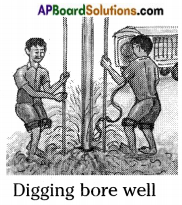2) Frictional force: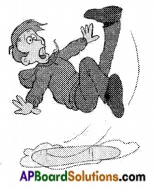3) Normal force: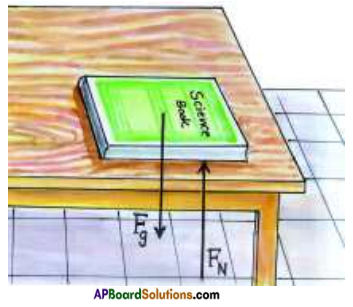4) Tension: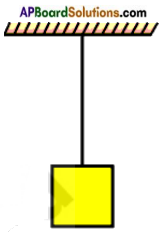II. Forces at distance (Field Forces)
1) Magnetic force: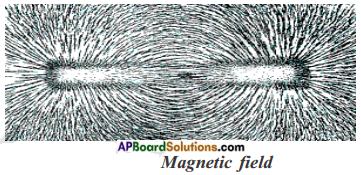2) Electrostatic force: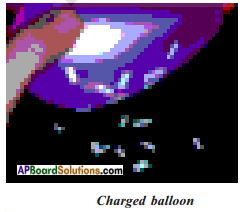3) Gravitational force: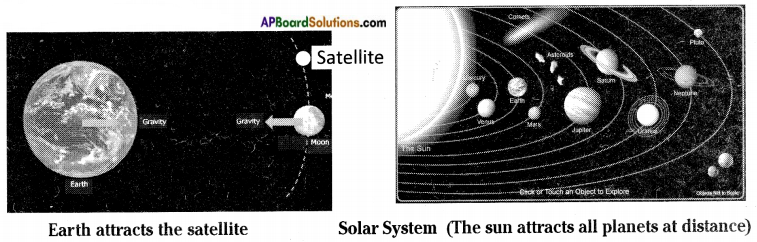Report: Forces are two types based on contact or non-contact between objects.I. Contact Forces : Force which results when there is a direct physical contact between two interacting objects is known as contact force. Contact forces are four types. They are :

1. Muscular Force: The force which we exert by using our body muscles is known as muscular force.
2. Frictional Force: Friction is the resistance to the movement of a body over the surface of another body.
3. Normal Force: The force that a solid surface exerts on any object in the normal direction is called normal force.
4. Tension: The lightness of slope or string when we try to stretch a rope or string is called tension.

II. Forces at distance (Field forces): The force which occurs without any physical con¬tact between two objects is known as a force at distance or field force.
Forces at distance are three types. They are:

1. Magnetic Force : The force existing between two magnetic poles of two different magnets is called magnetic force. The force is also exists between magnet and magnetic material.
2. Electrostatic Force : The force exerted by a charged body on another charged body is known as electrostatic force.
3. Gravitational Force : The force of attraction exists between any two bodies (or masses) everywhere in the universe is known as gravitational force.

Question 3.
When you push a heavy object, it doesn’t move. Explain the reason in terms of net force.
There are four forces acting on the heavy table. They are normal force (upwards), gravitational force (downwards), push and friction (opposite to push).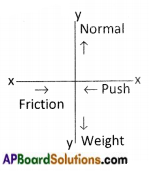Let’s take
Normal force = N (- ve); Gravitational Force = W (downwards); Push = F1
Friction = F2 (- ve) [more]
∴ Net force in X – direction = Push – Friction = F1 – F2 = – ve
Net force in Y – direction = N – W = – ve
As the net force in Y – axis is more you are unable to move the heavy object.

Question 4.
Some forces are acting without any contact of surfaces. Explain.
Some forces like magnetic force, electrostatic force and gravitational force act on other bodies without any contact of surfaces. This property is called the field force.
As far as magnetic force concern, the magnets are surrounded by the magnetic lines of force which provide the magnets attractive and repelling forces. The same poles repel and the different poles attract.
The electrostatic forces are exerted by a charged body on another charged or uncharged body. The charged body induces an opposite charge on the uncharged body and it gets attracted by the body. Here, the two bodies are at distance before the electrostatic forces are exerted.
The earth attracts everybody even though they are at some distance. This force is called gravitational force or weight of the body. It varies at different places.Question 5.
In the following situations identify the agent exerting the force and the object on which its acts. State the effect of force in each case.
a) A batsman hitting a cricket ball
b) Pressing a lump of dough
c) Taking out paste from a tooth paste
d) A horse pulling a cart

 Agent exerting force Object on which it acts Form of effect Muscles Cricket ball Change in speed and direction Fingers Dough Change in shape Fingers Tooth paste Change in shape Horse Cart Change in speed

### 8th Class Physical Science 1st Lesson Force Important Questions and Answers

Question 1.
A fruit is falling from a tree. Which force acts on the fruit?
Gravitational force acts on the fruit, which is from a tree.

Question 2.
Find the net force from the following diagrams.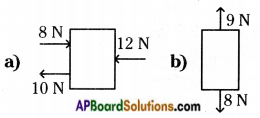a) Net force = + 12N + 10N – 8N = + 22N – 8N = 14 N
b) Net force = +9N – 8N = 1N

Question 3.
If you bring a rubbed balloon near the pieces of paper, what do you observe ?
Rubber balloon attracts pieces of paper. It is electrostatic force.

Question 4.
From the above diagram, calculate the net force acting on a body.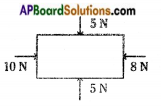Net force in vertical direction = 5N – 5N = 0
Net force in horizontal direction = 10N – 8N = 2NQuestion 5.
Find the net forces from the following free body diagram (FED).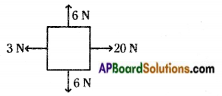i) Net force in the vertical direction = + 6N – 6N = 0N
ii) Net force in the horizontal direction = + 20N – 3N = 17N.

Question 6.
What will happen if gravitational force disappears from the Earth? Explain.
If gravitational force disappears from the earth,
i) No atmospheric gases are found around the earth.
ii) Objects does not fall down on the earth.
iii) Human beings, animals can fly easily in the sky.
iv) We cannot find the weight of the objects.

Question 7.
Define contact force and force at a distance, give two examples for each type of force.